# Common Core: High School - Geometry : Rotations and Reflections of Rectangles, Parallelograms, Trapezoids, and Regular Polygons: CCSS.Math.Content.HSG-CO.A.3

## Example Questions

← Previous 1

### Example Question #1 : Rotations And Reflections Of Rectangles, Parallelograms, Trapezoids, And Regular Polygons: Ccss.Math.Content.Hsg Co.A.3

Determine whether the statement is true or false:

The following image can be divided multiple ways to result in a reflected image.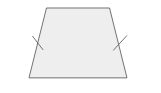True

False

False

Explanation:

Looking at the statement and the image given, it is seen that the object is a trapezoid.

A trapezoid has two bases of differing lengths and two side pieces of equal length in this particular case.For an object to be divided into images that can be reflected onto one another, the images must be identically mirrored.

To identify the possible solutions, draw the lines of symmetry.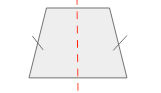Only one line of symmetry could be drawn to allow for two images of the trapezoid to be reflected over that line. The trapezoid cannot be divided in any other way that will result in a reflected image.

Therefore, the original statement is false.

### Example Question #21 : High School: Geometry

Given a hexagon, how many lines of symmetry exist?

Six

Two

Twelve

Three

Four

Six

Explanation:

Recall that a hexagon is a six sided figure. Now recall that a line of symmetry creates two mirrored, identical figures which is also known as a reflection.

Knowing these two pieces of information draw the image of the hexagon and the lines of symmetry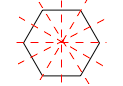Counting up these lines results 6 lines of symmetry.

### Example Question #22 : Congruence

A cylinder is composed of two circular bases. For one base to be carried onto the other, what geometric transformation must occur?

Translation

Rotation

All of the answers are correct.

Rotation and Translation

Extension

Translation

Explanation:

Since a cylinder is composed of two, identical circular bases that are separated by the height of the cylinder, the transformation that must occur to have one carried onto the other is a translation. Recall that a translation is the sliding of an object without changing its size or shape. Therefore, for the one base to be carried onto the other, translation is the geometric transformation that must occur.

### Example Question #1 : Rotations And Reflections Of Rectangles, Parallelograms, Trapezoids, And Regular Polygons: Ccss.Math.Content.Hsg Co.A.3

If the rectangle is reflected across the x-axis, what is the resulting image?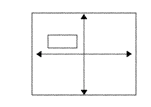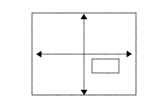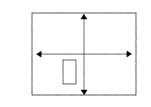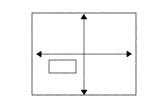Explanation:

In order to create the resulting image of a reflection first recall what a reflection is.

Reflection: To flip the orientation of an object over a specific line or function.

In this specific situation the line of reflection is the x-axis.

The original image is,When the image is reflected across the x-axis result in,### Example Question #23 : Congruence

Given a trapezoidal based prism how can one base be carried onto the other?

Reflection

Extension

Rotation

Translation

Translation

Explanation:

Since a trapezoidal based prism is composed of two, identical trapezoidal bases that are separated by the height of the prism, the transformation that must occur to have one base carried onto the other is a translation. Recall that a translation is the sliding of an object without changing its size or shape. Therefore, for the one base to be carried onto the other, translation is the geometric transformation that must occur.

### Example Question #24 : Congruence

Determine whether the statement is true or false:

Reflections and rotations never result in the same image.

False

True

False

Explanation:

To determine whether the statement is true or false, identify any example that would make the statement false.

"Reflections and rotations never result in the same image."

Imagine a square that exists in quadrant two. When this image is reflected across the y-axis it is still a square that is now in quadrant one. When the square from quadrant two is rotated around the origin, it results in the same image in quadrant one. Therefore, it is possible for a reflection and rotation to result in the same image.

Thus, the statement "Reflections and rotations never result in the same image." is false.

### Example Question #1 : Rotations And Reflections Of Rectangles, Parallelograms, Trapezoids, And Regular Polygons: Ccss.Math.Content.Hsg Co.A.3

If the rectangle is rotated clockwise around the origindegrees, what is the resulting image?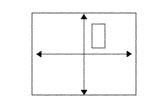Explanation:

To rotate the rectangular object around the origin, first recall the definition for a rotation and origin.

Rotation: To rotate an object either clockwise or counter clockwise around a center point. In this particular case the center point is the origin or the pointwhere the x and y axis intersect.

Looking at the original image and making one 90 degree rotation around the origin results in the following.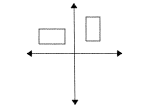When rotating, the bottom right point will become the bottom left point, the top right point becomes the bottom right point, the left bottom point becomes the top left point, and the left top point becomes the top right point.

Now, rotating it anotherdegrees to get to thedegree rotated image, results in the following### Example Question #1 : Rotations And Reflections Of Rectangles, Parallelograms, Trapezoids, And Regular Polygons: Ccss.Math.Content.Hsg Co.A.3

If the rectangle is rotated clockwise around the origindegrees, what is the resulting image?Explanation:

To rotate the rectangular object around the origin, first recall the definition for a rotation and origin.

Rotation: To rotate an object either clockwise or counter clockwise around a center point. In this particular case the center point is the origin or the pointwhere the x and y axis intersect.

Looking at the original image and making one rotation around the origin results in the following.When rotating, the bottom right point will become the bottom left point, the top right point becomes the bottom right point, the left bottom point becomes the top left point, and the left top point becomes the top right point. The visual representation for this rotation is as follows.### Example Question #9 : Rotations And Reflections Of Rectangles, Parallelograms, Trapezoids, And Regular Polygons: Ccss.Math.Content.Hsg Co.A.3

If the rectangle is rotated clockwise around the origindegrees, what is the resulting image?Explanation:

To rotate the rectangular object around the origin, first recall the definition for a rotation and origin.

Rotation: To rotate an object either clockwise or counter clockwise around a center point. In this particular case the center point is the origin or the pointwhere the x and y axis intersect.

Looking at the original image and making onedegree rotation around the origin results in the following.When rotating, the bottom right point will become the bottom left point, the top right point becomes the bottom right point, the left bottom point becomes the top left point, and the left top point becomes the top right point.

Now, rotating it anotherdegrees to get to thedegree rotated image, results in the followingFrom here one final rotation must occur to reachdegrees.### Example Question #1 : Rotations And Reflections Of Rectangles, Parallelograms, Trapezoids, And Regular Polygons: Ccss.Math.Content.Hsg Co.A.3

If the rectangle is rotated clockwise around the origindegrees, what is the resulting image?Explanation:

To rotate the rectangular object around the origin, first recall the definition for a rotation and origin.

Rotation: To rotate an object either clockwise or counter clockwise around a center point. In this particular case the center point is the origin or the pointwhere the x and y axis intersect.

Looking at the original image and making one 90 degree rotation around the origin results in the following.When rotating, the bottom right point will become the bottom left point, the top right point becomes the bottom right point, the left bottom point becomes the top left point, and the left top point becomes the top right point.

Now, rotating it anotherdegrees to get to thedegree rotated image, results in the followingFrom here another rotation must occur to reachdegrees.Lastly, complete one moredegree rotation to land at thedegree mark. Notice that rotating 360 degrees lands the image back at its original spot.← Previous 1

### All Common Core: High School - Geometry Resources